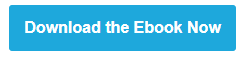# Support Vector Machines Succinctly released## About Support Vector Machines Succinctly

While I was working on my series of articles about the mathematics behind SVMs, I have been contacted by Syncfusion to write an ebook in their "Succinctly" e-book series. The goal is to cover a particular subject in about 100 pages. I gladly accepted the proposition and started working on the book.

I took me almost one year to complete writing this ebook. Hundred of hours spent reading about Support Vector Machines in several books and papers, trying to figure out the complex things in order to give you a clear view. And just as much drawing schemas and writing code.

## What you will find in this book?

• A refresher about some prerequisites to understand the subject more easily
• An introduction with the Perceptron
• An explanation of the SVM optimization problem
• How to solve the SVM optimization problem with a quadratic programming solver
• A description of kernels
• An explanation of the SMO algorithm
• An overview of multi-class SVMs
• A lot of Python code to show how it works (everything is available in this bitbucket repository )

I hope this book will help all people strugling to understand this subject to have a fresh and clear understanding.

## Is it hard to read?

My goal was to try to keep the book as easy to understand as possible. However, because of the space available, I was not able to go into as much details as in this blog.

Chapter 4 about the optimization problem will probably be the most challenging to read. Do not worry if you do not catch everything the first time and feel free to use it as a base to dive deeper into the subject if you wish to understand it fully.

If you struggle on some part, you can ask your question on stats.stackexchange if it is about Machine Learning or on math.stackexchange if it is about the mathematics. Both communities are great.

## What now?

If you read the book I would love to hear your feedback!

### 28 thoughts on “Support Vector Machines Succinctly released”

1.amazing.
thank u sir

2.Alexandre,

thanks for the great book on svm. May I have a question about Fig26 on page 42? I do not understand why the examples are incorrectly classified. As the hyperplane is x1-x2 = 0. and the red point (8, 6) get a predicted value +1 as its value is 2, which is correctly classified. Any explanation is quite appreciate. Thanks a lot.

•Hello Alice,

Yes indeed, it looks like there is a typo, and that the two figures are switched.
Figure 26 correctly classifiy the data, and Figure 25 does not.

Thanks for message!

I used the code below to check:
``` import numpy as np```

``` # We associate each vector x_i with a label y_i, # which can have the value +1 or -1 # (respectively the triangles and the stars in Figure 13). # triangle = +1 # stars = -1 # Hyperplane of figure 25 w = np.array([-1, -1]) bias = 12 print(np.dot([4,6], w) + bias) # returns 2 so gets classified as +1 print(np.dot([8,6], w) + bias) # returns -2 so gets classified as -1 # Hyperplane of figure 26 w = np.array([1, -1]) bias = 0 print(np.dot([4,6], w) + bias) # returns -2 so gets classified as -1 print(np.dot([8,6], w) + bias) # returns 2 so gets classified as +1 # [8,6] is a triangle, so its value is +1, # it is correctly classified by figure 26 # and it is incorrectly classified by figure 25 ```

3.Dear Alexandre KOWALCZYK
I couldn't understand how to say thank you. The way you teach SVM is really fantastic. I didn't find any other tutorial better than your post. I also want to learn others like logistic regression , random forest etc. Do you have also tutorial for those. I am really interested.

Thank you. Wish you a happy life.

4.I think one of the most difficult things is to be simple with simple things, and simple with difficult things. This is an art... and you are an artist 😉

5.Hi Alexandre,
really enjoyed your book so far, definitely the best tutorial on svm I have seen.
But on page 59, I simply can not understand, how to compute the vector w.
I know that xi is a particular training example and is of dimension (m x 1), where m = number of training examples. The vector should be a (n x 1) dimensional vector, where n = number of variables, right?
If so, I can not understand how to compute the vector w. For example, how does the first element of w, w1, get computed?
Thanks a lot.

•```def compute_w(multipliers, X, y): return np.sum(multipliers[i] * y[i] * X[i] for i in range(len(y))) ```